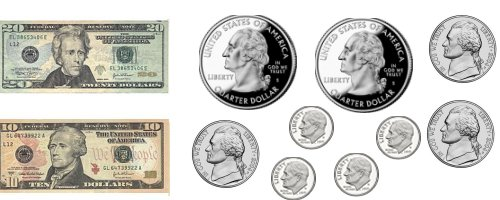Parents and Teachers: Support Ducksters by following us onor.

Math >> Money and Finance

# Money Math

## Making Change

It's good to know how to make change whether you work in a store or are just shopping. People can make mistakes and it's a good idea to make sure that you get the right amount of change when shopping.

Subtracting

Making change is really just subtracting. If you can subtract using decimals, you can make change. You don't want to have to get out a pencil and paper or your calculator in the store, however, so it's a good idea to know how to make change in your head.

Tips to Counting Change

It's always a good idea to figure your change when at the store. You can even make a game of it. Try to figure out the change you should get before the cash register gives you an answer. Then, once you get your change, count it out. You can double check your change by counting out the change you received and adding it to the price of the bill. This should all add up to the amount you paid.

Formula for Checking Change

Change + Bill = What you Paid

So if the bill was \$8 and you gave the cashier a \$10, then you got \$2 in change. You can check your change using the formula. It would look like:

\$2 + \$8 = \$10

This sounds really simple, but doing this simple check will help you get better at counting your change and will often catch mistakes made by the cashier.

Example Problem 1

The price of an item is \$17. You give the cashier a \$20 bill. What is the change?

Answer:

20 - 17 = \$3.

Example Problem 2

The Price of an item is 12.75. You give the cashier a \$10 and a \$5 bill. What is the change?

Answer:

10 + 5 = \$15

15.00 - 12.75 = \$2.25

What if you didn't have a calculator? What is an easy way to think of this problem?

First you could subtract the \$12 from the \$15.

\$15 - \$12 = \$3

Then you could subtract the 75 cents from the \$3.

\$3 minus 75 cents = two dollars and 25 cents or \$2.25

Example Problem 3

The price of an item is \$129.43. You give the cashier 7 twenties and two quarters. What is your change?

Answer:

First let's figure the dollars part:

7x \$20 = \$140, so we subtract 129 from 140 = 140 - 129 = \$11

Next, let's figure the cents part:

Two quarters = 50 cents, we subtract 43 cents from 50 cents = 50 - 43 = 7 cents

We put the dollars and cents together to get the answer:

11 dollars and 7 cents = \$11.07

Example Problem 4

The price of the item is 26 dollars and 45 cents. You give them the money shown in the picture below. What is the change?First lets add up the money in the picture:

There are \$30 in bills and 105 cents or \$1.05 in coins. The total is \$31.05.

We'll subtract the dollars first:

31 - 26 = \$5

Now we subtract the cents:

5 cents minus 45 cents. We don't have enough cents. So let's take one of the dollars and convert it to cents. This gives us 105 cents. Remember now we only have 4 dollars.

105 cents - 45 cents = 60 cents

We should get 4 dollars and 60 cents in change.

Learn More about Money and Finance:

Note: This information is not to be used for individual legal, tax, or investment advice. You should always contact a professional financial or tax advisor before making financial decisions.
Math >> Money and FinanceAbout Ducksters Privacy Policy

Follow us onorThis site is a product of TSI (Technological Solutions, Inc.), Copyright 2019, All Rights Reserved. By using this site you agree to the Terms of Use.